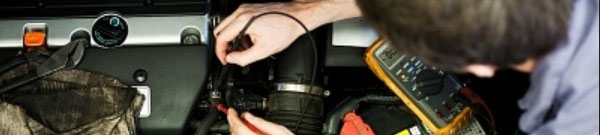# Types of Planetary Gear UnitThere are various types of planetary gear units. Here, typical planetary gear units are:

1. 3-speed + O/D type (FF vehicles)

By combining a 3-speed planetary gear unit with an O/D gear unit, four forward gear ratios and one reverse gear ratio are generated.

2. 3-speed + O/D type (FR vehicles)

3-speed x O/D type (FR vehicles)

The O/D gear unit for FR vehicles is placed between the torque converter and 3-speed planetary gear unit, which location is different from that of FF vehicles.

However the configuration is the same as for FF vehicles. Therefore four forward gear ratios and one reverse gear ratio are generated. Also, in the A350, the 1st gear and the O/D gear are combined to produce the 2nd gear. In this way, five forward gear ratios and one reverse gear ratio are generated.

3. 4-speed + O/D type (FR vehicles)

A center planetary gear is placed between the front planetary gear and the rear planetary gear. By combining these with the O/D gear unit, five forward gear ratios and one reverse gear ratio are generated.

4. 5-speed type (FR vehicles)

A center planetary gear is placed between the front planetary gear and the rear planetary gear. Also, the front planetary gear has two pinion gears arranged between the ring gear and the sun gear. By combining these planetary gear unit, five forward gear ratios and one reverse gear ratio are generated.

5. 4-speed CR-CR type (FF vehicles)

Four forward gear ratios and one reverse gear ratio can be generated with two planetary gears. A CR-CR planetary gear is a type of planetary gear unit that joins the front and rear planetary carriers to the ring gear.

6. 4-speed ravigneaux type (FF vehicles)

A long pinion and short pinion are placed between the ring gear and the front sun gear. The long pinion also meshes with the rear sun gear. Four forward gear ratios and one reverse gear ratio can be generated.

7. 3-speed + U/D type (FF vehicles)

One planetary gear is placed on the counter shaft. This operates as an “under-drive” reduction unit. The same as the 3-speed + O/D type, four forward gear ratios and one reverse gear ratio are generated. The gear ratio for the top gear is same as O/D for the total reduction ratio including the differential gear ratio.

8. 4-speed + U/D type (FF vehicles)

A 4-speed CR-CR type planetary gear unit is placed on the input shaft and an “under-drive” reduction unit is placed on the counter shaft. With these, five forward gear ratios and one reverse gear ratio are generated.